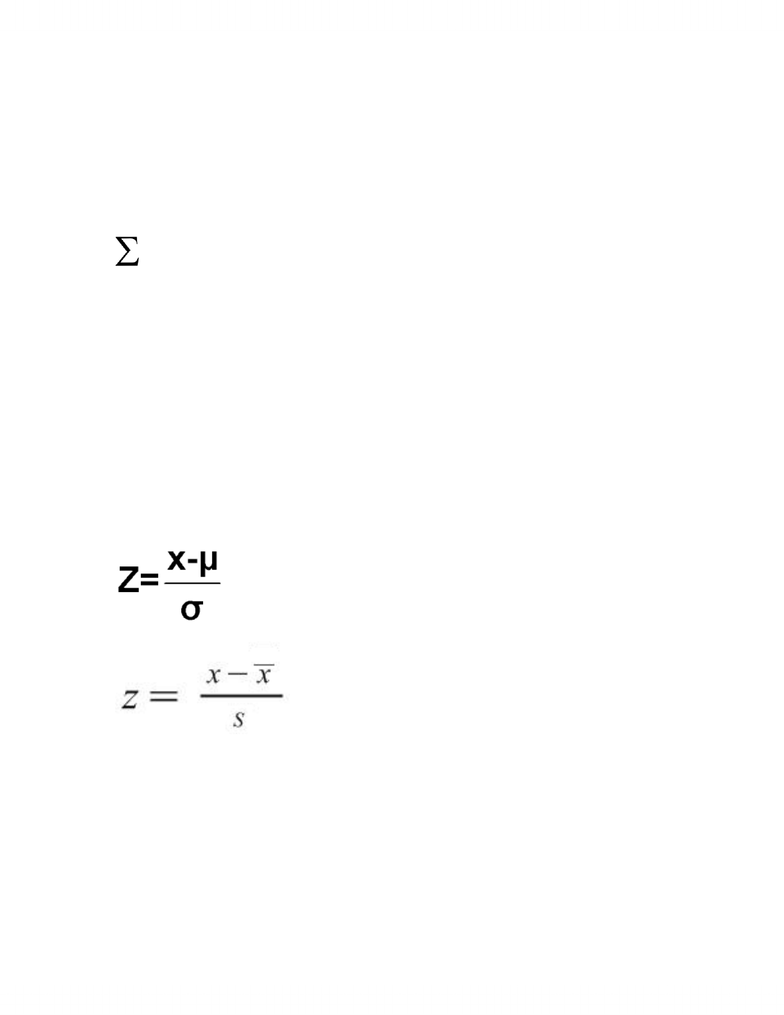Class Notes (1,100,000)
CA (620,000)
U of G (30,000)
SOAN (900)
SOAN 2120 (400)
Lecture

SOAN 2120 Lecture Notes - Variance, Standard Deviation, Statistical Significance

Department
Sociology and Anthropology
Course Code
SOAN 2120
Professor
David Walters

This preview shows half of the first page. to view the full 3 pages of the document.September 27, 2012
Population parameters the entire group we are interested in
Sample statistics a portion of the population
Central tendency mean, median mode
Mean average
Median middle number
Mode most occurring
X use value from data set
N number of values in your data set
Measures of variability - range, deviation, variance, standard deviation
Range = highest value lowest value
Deviation how far a measurement is form the mean/average of the set
- X minus the mean
Variance - to find sample variance sum of the squared of deviations divided by n
minus 1 from the number of values in a set
Standard deviation
Relative standing = z-score
Take ‘x’ substract mean and divide by standard deviation
POPULATION formula:
SAMPLE formula:
“s” is the standard deviation
the “x” is where you plug in the obersavtion
number… which is the number you see in the data set
the x with line over top is the mean
**NOTE THIS: population and sample formula are really the same. You still plug
everything in the same way.**
outlier just get rid of it… it’s that number that’s way off from the rest of the data
set
Understanding z-scores
You're Reading a Preview

Unlock to view full version